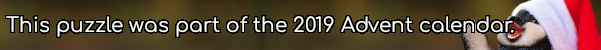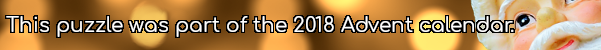mscroggs.co.uk
mscroggs.co.uksubscribe

# Puzzles

## 24 DecemberThere are six 3-digit numbers with the property that the sum of their digits is equal to the product of their digits. Today's number is the largest of these numbers.

## 22 DecemberIn bases 3 to 9, the number 112 is: $$11011_3$$, $$1300_4$$, $$422_5$$, $$304_6$$, $$220_7$$, $$160_8$$, and $$134_9$$. In bases 3, 4, 6, 8 and 9, these representations contain no digit 2.
There are two 3-digit numbers that contain no 2 in their representations in all the bases between 3 and 9 (inclusive). Today's number is the smaller of these two numbers.

## 17 DecemberEve picks a three digit number then reverses its digits to make a second number. The second number is larger than her original number.
Eve adds her two numbers together; the result is 584. What was Eve's original number?

## 1 DecemberIf you write out the numbers from 1 to 1000 (inclusive), how many times will you write the digit 1?

## Not Roman numerals

The letters $$I$$, $$V$$ and $$X$$ each represent a different digit from 1 to 9. If
$$VI\times X=VVV,$$
what are $$I$$, $$V$$ and $$X$$?

## 22 DecemberIn base 2, 1/24 is 0.0000101010101010101010101010...
In base 3, 1/24 is 0.0010101010101010101010101010...
In base 4, 1/24 is 0.0022222222222222222222222222...
In base 5, 1/24 is 0.0101010101010101010101010101...
In base 6, 1/24 is 0.013.
Therefore base 6 is the lowest base in which 1/24 has a finite number of digits.
Today's number is the smallest base in which 1/10890 has a finite number of digits.
Note: 1/24 always represents 1 divided by twenty-four (ie the 24 is written in decimal).

## 15 DecemberToday's number is smallest three digit palindrome whose digits are all non-zero, and that is not divisible by any of its digits.

## 9 DecemberToday's number is the number of numbers between 10 and 1,000 that contain no 0, 1, 2 or 3.

## Archive

Show me a random puzzle
▼ show ▼